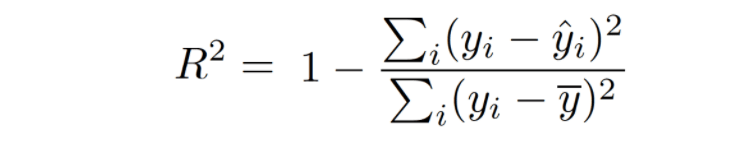Net-informations.com

# What is Adjusted R-Squared

Adjusted R-Squared is a modified form of R-Squared whose value increases if new predictors tend to improve models performance and decreases if new predictors does not improve performance as expected. R-squared is a comparison of Residual sum of squares (SSres) with total sum of squares(SStot). It is calculated by dividing sum of squares of residuals from the regression model by total sum of squares of errors from the average model and then subtract it from 1.Unlike R-squared, the Adjusted R-squared would penalize you for adding features which are not useful for predicting the target. It takes into account the number of independent variables used for predicting the target variable.where,

1. N = number of records in the data set.
2. p = number of independent variables.

For a simple representation, you can rewrite the above formula like the following:

Adjusted R-squared = 1 — (x * y)

where,

1. x = 1 — R Squared
2. y = (N-1) / (n-p-1)

Adjusted R-squared can be negative when R-squared is close to zero.
Adjusted R-squared value always be less than or equal to R-squared value.

## Adjusted R-squared using sklearn.metrics

net-informations.com (C) 2022    Founded by raps mk